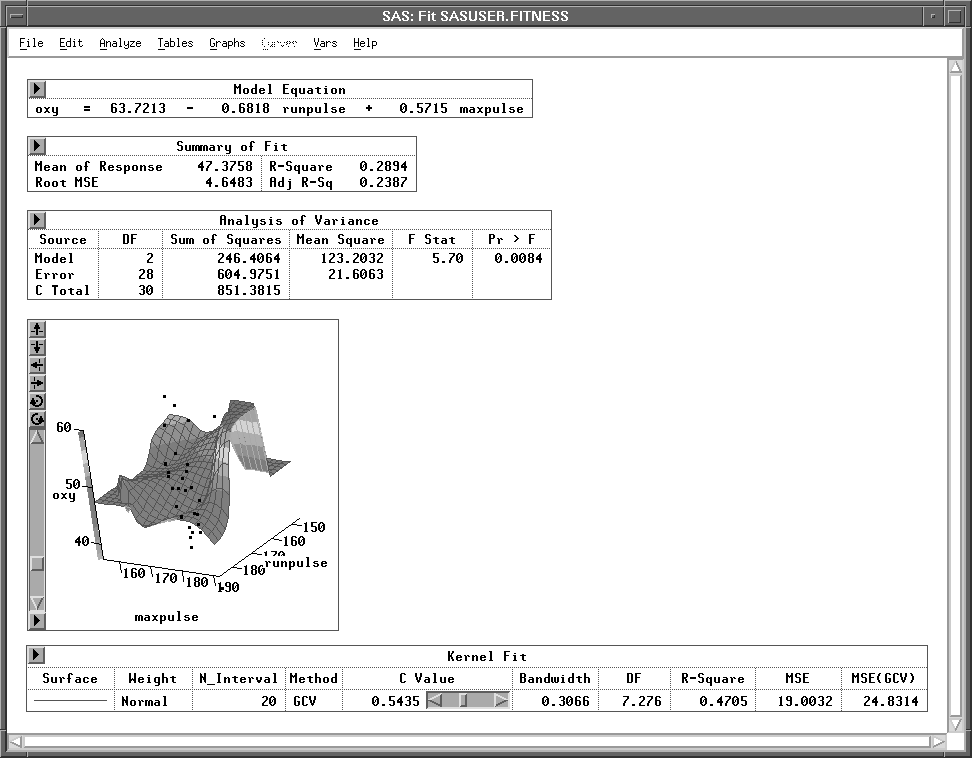Previous Page Next Page
 Fit Analyses

# Fit Analyses

Choosing Analyze:Fit ( Y X ) gives you access to a variety of techniques for fitting models to data. These provide methods for examining the relationship between a response (dependent) variable and a set of explanatory (independent) variables.

You can use least-squares methods for simple and multiple linear regression with various diagnostic capabilities when the response is normally distributed.

You can use generalized linear models to analyze the data when the response is from a distribution of the exponential family and a function can be used to link the response mean to a linear combination of the explanatory variables.

You can use spline and kernel smoothers for nonparametric regression when the model has one or two explanatory variables.Figure 39.1: Fit Analysis

#### References

 Previous Page Next Page Top of Page